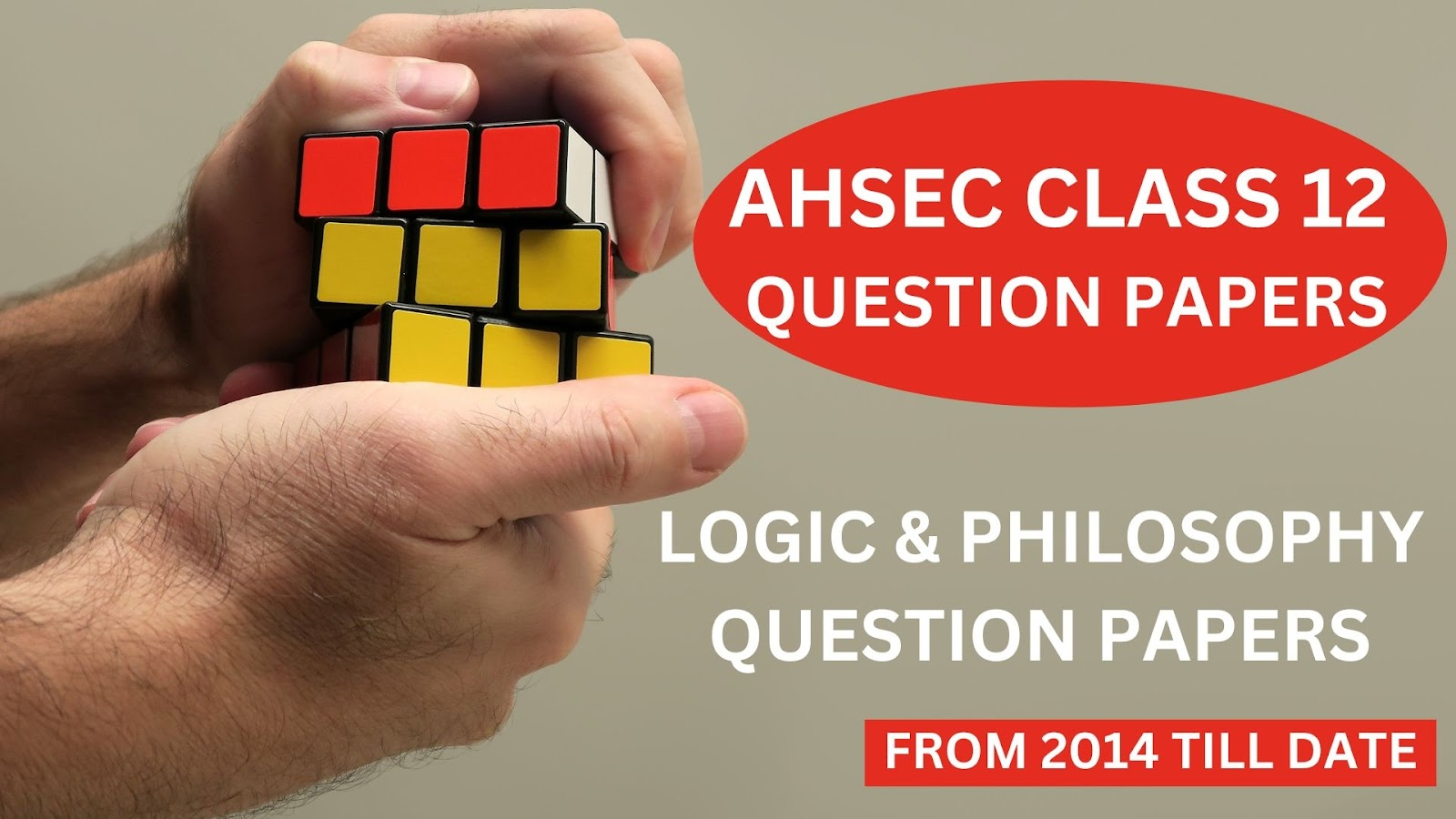# Logic and Philosophy Question Paper' 2019 [AHSEC Class 12 Question Papers]## Logic and Philosophy Question Paper' 2019AHSEC Class 12 Question PapersFull Marks: 100Pass Marks: 30Time: 3 hours

The figures in the margin indicate full marks for the questions
1. (a) What is the main subject matter of Logic?                         1
(b) Is the conclusion of Unscientific Induction certain?                   1
(c) Is there ‘inductive leap’ in Perfect Induction?                       1
(d) The Paradox of Induction is connected with the Principle of the Uniformity of Nature. Is it true?                     1
(e) Which is the first stage of hypothesis suggested by Miss’s definition of hypothesis?                                                             1
(f) What is the meaning of ‘vera causa’?                   1
(g) What is the exact object of moral judgement?               1
(h) Name the theory which states that the external world can be directly known.                                                         1
(i) Is the conclusion of Method of Agreement certain?                1
(j) Ethics is a positive science. Is it true?                                                      1
(k) From which Sanskrit root the word ‘Dharma’ is derived?        1
(l) Which principle of elimination forms the basis of Method of Agreement?       1
2. State two points of differences between deduction and induction.                            2
3. Why the law of causation is called the formal ground of induction?                   2
4. Define Scientific Induction.                                              2
5. State any two qualitative marks of causation.                 2
Or
Define conjunction of causes with an example.                                2
6. How many types of verification are there in a hypothesis and what are these?      2
Or
What do you mean by analogical hypothesis?                    2
7. Write two criticisms of Native Realism.                     2
8. Give a definition of Idealism in your own words.                         2
9. Distinguish between fallacy of non-observation and fallacy of mal-observation.       2
10. Write two advantages of observation as the material ground of Induction.                                    1+1=2
11. Why the Idealism of Leibnitz is called Pluralistic Idealism?               2
12. Define negative condition with the help of a suitable example.                1+1=2
13. Write the name of one primary and one secondary quality of an object.                                         1+1=2
14. State the qualitative marks of a cause.                        4
Or
What do you mean by plurality of causes? Why plurality of causes is not scientifically acceptable?2+2=4
15. Do you support Native Realism? Give reasons in support of your view.         1+3=4
16. What is ‘Crucial Instance’? Explain.                           4
17. State two advantages and two disadvantages of the Method of difference.     2+2=4
Or
Write two advantages and two disadvantages of the Method of Agreement.          2+2=4
18. Briefly express any four characteristics of Scientific Realism.                      4
19. Name the philosopher who made the statement ‘Esse Est Percipi’. Explain the meaning of the statement.    1+3=4
Or
Do you think that Hegel’s idealism tries to reconcile idealism and realism? Explain.
20. Explain briefly the Dharma and Artha as Purusarthas.            2+2=4
Or
State four important aspects with which Ethics deals.              4
21. Write a note on Plato’s Idealism.                  4
22. Write briefly the nature of religion.                        4
23. Briefly explain the four stages of hypothesis.              4
24. State any three similarities and any three dissimilarities between Unscientific Induction and Analogy.              3+3=6
Or
Define Unscientific Induction and state its main characteristics.
25. Define the Joint Method of Agreement and Difference with concrete example. State two advantages and two disadvantages of this method                   2+2+2=6
Or
Explain the Method of Residues with example.            6
26. Explain Aristotle’s view on causation.                           6
Or
Explain the Law of Causation as one of the formal grounds of Induction.              6
27. “Religion and Morality are mutually dependable” – Discuss it.             6
Or
What do you mean by Religion? Which is the most acceptable definition of Religion? Explain two characteristics of Religion.                      2+2+2=6